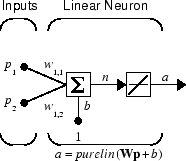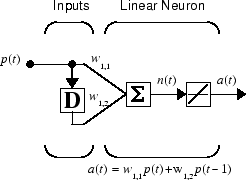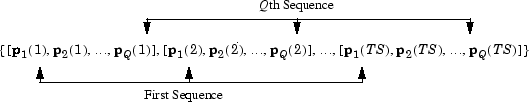@devilogic 2016-08-02T06:11:13.000000Z 字数 2280 阅读 981

# 理解神经网络工具箱的数据结构

matlab

## 仿真并行输入的静态网络net = linearlayer;                  % 使用线性函数net.inputs{1}.size = 2;             % 有两个输入单元net.layers{1}.dimensions = 1;       % 仅有一个隐藏层，这层只有一维

net.IW{1,1} = [1 2];                % 输入权重两个net.b{1} = 0;                       % 偏置值为0

P = [1 2 2 3; 2 1 3 1]              % 单位是以列向量

A = net(P)A =     5    4    8    5

## 仿真串行输入的动态网络net = linearlayer([0 1]);net.inputs{1}.size = 1;         % 输入单元为1net.layers{1}.dimensions = 1;net.biasConnect = 0;            % 偏置值为0

net.IW{1,1} = [1 2];

P = {1 2 3 4};          % 这里是cell数组类型

A = net(P)A =        

## 仿真并行输入的动态网络

P = [1 2 3 4];          % 这里是向量类型

A = net(P)A =     1    2    3    4

P = {[1 4] [2 3] [3 2] [4 1]};

A = net(P);

A = {[1 4] [4 11] [7 8] [10 5]}

$p$就好比是一个人，$p_1(TS)$好比这个人在$TS$时间点的行为值$1$$p_2(TS)$表示这个人在$TS$时间点的行为值$2$• 私有
• 公开
• 删除